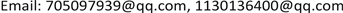JSTAJournal of Sensor Technology and Application2331-0235Scientific Research Publishing10.12677/JSTA.2020.84013JSTA-37922JSTA20200400000_30522097.pdf工程技术 空心线圈传感器的变阻尼标定方法研究 Research on Variable Damping Calibration Method of Air-Core Coil Sensor 21浩文1314林山2121凡君21志红51国超21云南电网有限责任公司电力科学研究院，云南 昆明重庆大学，重庆null云南电网有限责任公司红河供电局，云南 红河州长江师范学院，重庆280920200804115122© Copyright 2014 by authors and Scientific Research Publishing Inc. 2014This work is licensed under the Creative Commons Attribution International License (CC BY). http://creativecommons.org/licenses/by/4.0/

Research on Variable Damping Calibration Method of Air-Core Coil Sensor

Bo Li1, Haowen Wang2, Ying Shen3, Linshan Zhang1, Min Cao1, Fanjun Hu1, Zhihong Fu4, Guochao Qian1

1Electric Power Research Institute of Yunnan Power Grid Co., Ltd., Kunming Yunnan

2Yangtze Normal University, Chongqing

3Honghe Power Supply Bureau of Yunnan Power Grid Co., Ltd., Honghe Yunnan

4Chongqing University, ChongqingReceived: Sep. 9th, 2020; accepted: Sep. 21st, 2020; published: Sep. 28th, 2020ABSTRACT

The inductive air-core coil sensor is an important time-varying magnetic field measurement tool. Due to the inherent inductance and distributed capacitance of the coil, the output voltage of the coil cannot accurately follow the sudden induced voltage. This phenomenon is called the transition process of the coil. The existing correction methods can only be completed by the laboratory, and it is difficult to calibrate the parameter disturbance caused by the field environment. This paper takes the air-core coil sensor used in transient electromagnetic detection as an example, and proposes a scheme to eliminate the transition process by using the transfer function. By analyzing the equivalent circuit of the receiver coil, a variable damping coil calibration method suitable for solving the parameters of the receiver coil in the field environment is proposed. The device has a simple form and high reliability, which meets the needs of field calibration in the construction environment.

Keywords:Transient Electromagnetic, Air-Core Coil Sensor, Transition Process

Copyright © 2020 by author(s) and Hans Publishers Inc.

This work is licensed under the Creative Commons Attribution International License (CC BY 4.0).

http://creativecommons.org/licenses/by/4.0/1. 引言

2. 空心线圈的过渡过程

H ( s ) = u ( s ) ε ( s ) = 1 s 2 L C + s ( L R b + R C ) + R + R b R b (1)

ε ( t ) L C = d 2 u ( t ) d t 2 + 2 δ d u ( t ) d t + ω p 2 u ( t ) (2)

ξ = δ 1 ω p = R b R C + L 2 L C R b ( R + R b ) (3)

R 0 = L R C + 2 L C (4)

3. 变阻尼标定法

∬ v ( t ) d t 2 = τ l τ c α u ( t ) + ( τ l + τ c ) ∫ u ( t ) d t + ( 1 + α ) ∬ u ( t ) d t 2 (5)

0.8倍关系选取三个阻尼电阻值，获得三个参数方程。

{ V ( t ) = m 1 × u 1 ( t ) + m 2 × ∫ u 1 ( t ) d t + ( 1 + α ) × ∬ u 1 ( t ) d t 2 V ( t ) = m 1 × u 2 ( t ) + m 2 × ∫ u 2 ( t ) d t + ( 1 + α ) × ∬ u 2 ( t ) d t 2 V ( t ) = m 1 × u 3 ( t ) + m 2 × ∫ u 3 ( t ) d t + ( 1 + α ) × ∬ u 3 ( t ) d t 2 (6)

u 2 ( t ) 和 u 3 ( t ) 可以通过A/D采样直接得到。求解方程组(6)便可以确定参数 m 1 和 m 2 ，进而获取线圈的传递函数 H ( s ) 。

η = max { | L − L c t L | ∗ 1000 | C − C c t C | ∗ 1000 ‰ ‰ (7)

4. 讨论4.1. 采样率对结果的影响

4.2. 阻尼电阻R<sub>b</sub>对结果的影响

The influence of sampling rate on parameter solution error rat

The relationship between parameter error rate and tolerance of damping resistanc
Rb数列公差−0.1 * R0−0.2 * R0−0.4 * R0

4.3. 积分区间对结果的影响

The influence of the integration starting point on the error rate of parameter solutio

5. 实验结果

6. 小结